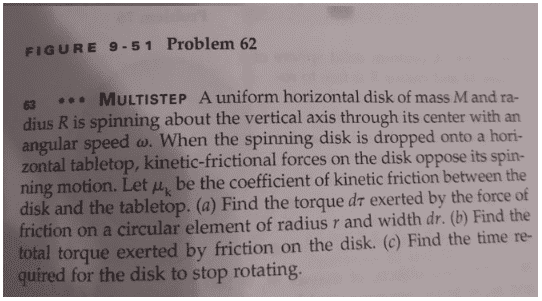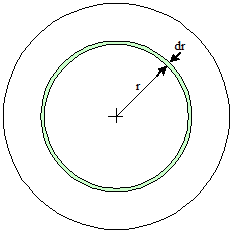# Confusing Derivation of torque acting on disk question

• Bonbon32
In summary, when the disk is on the tabletop, it exerts a force on the table surface. This force is given by the product of the disk's mass and the pressure it produces.f

#### Bonbon32

Homework Statement
A disk of mass M and angular speed w falls into table top. The kenetic coffienet of friction from table top is meu. Create an equation for dt=dr *F. Using this information.
Relevant Equations
Iaplha = torque
Fr = torqueNot sure how they obtained an answer of (2meukenetic *Mg/R^2)*r^2 dr = dt
When they say disk I am assuming it's not ring shaped. So since F * Dr= DT where I guess F acting on a small point on the disc is constant from the table top. And Dr is the variable distance from a random small point from the axis which summed together times force give total torque. I plug in F friction using mgmeu. Not to sure where to go from there.

#### Attachments

Last edited by a moderator:
The disk as a whole, lying on the tabletop, exerts a force ##M g## on the table surface, right? Assuming that the weight is evenly distributed over the entire disk-table interface, that means that the disk presents a pressure of ##Mg/\pi R^2##.

A given circular element of the disk will have an area associated with it (##\pi r^2 dr##). What is the force associated with that area element (you've got pressure and area)? Work out the ##d\tau## that that circular element will provide.

The disk as a whole, lying on the tabletop, exerts a force ##M g## on the table surface, right? Assuming that the weight is evenly distributed over the entire disk-table interface, that means that the disk presents a pressure of ##Mg/\pi R^2##.

A given circular element of the disk will have an area associated with it (##\pi r^2 dr##). What is the force associated with that area element (you've got pressure and area)? Work out the ##d\tau## that that circular element will provide.
Makes sense so (meu mg/R^2 )* r^2 *Dr which gives me a torque, not sure where they got the 2 from? Which is on the numerator next to the meu value. Finally got one more question if you can bear with me. Would the meu mg the friction be dF instead as we are taking only a small circular position about the rotating axis? So it becomes meu*dm*g ? If we aren't assuming anything.

Makes sense so (meu mg/R^2 )* r^2 *Dr which gives me a torque, not sure where they got the 2 from?
What's the area of the circular element of radius r and width dr?Would the meu mg the friction be dF instead as we are taking only a small circular position about the rotating axis? So it becomes meu*dm*g ?
Force is pressure times area. so the frictional force contribution for a given area element becomes ##dF = P \times dA##, where dA is the differential area element.

By the way, you can find a table of mathematical letters and symbols in the edit window top bar menu if you click on the ##\sqrt{x}## icon. There you will find, for example, the Greek letter μ. You don't need to call it "meu", if you can use the actual letter.

 Added an image to clarify what a "circular element" refers to.

Last edited:
•Bonbon32